- 11 minutes read

# Why Should I Learn SQL Window Functions?

SQL has been around for more than 25 years. Its basic syntax – like SELECT, WHERE, GROUP BY, HAVING, and ORDER BY – is well known. But is there something besides traditional SQL? Can you go beyond the basics and improve your skills?

The answer to this question is yes. There is a modern version of SQL. In this article, we are going to dive into the modern version and learn about SQL window functions. If you are an advanced beginner who wants to analyze data with the help of SQL’s window functions (also called analytic functions), this article is for you.

### A Brief History of SQL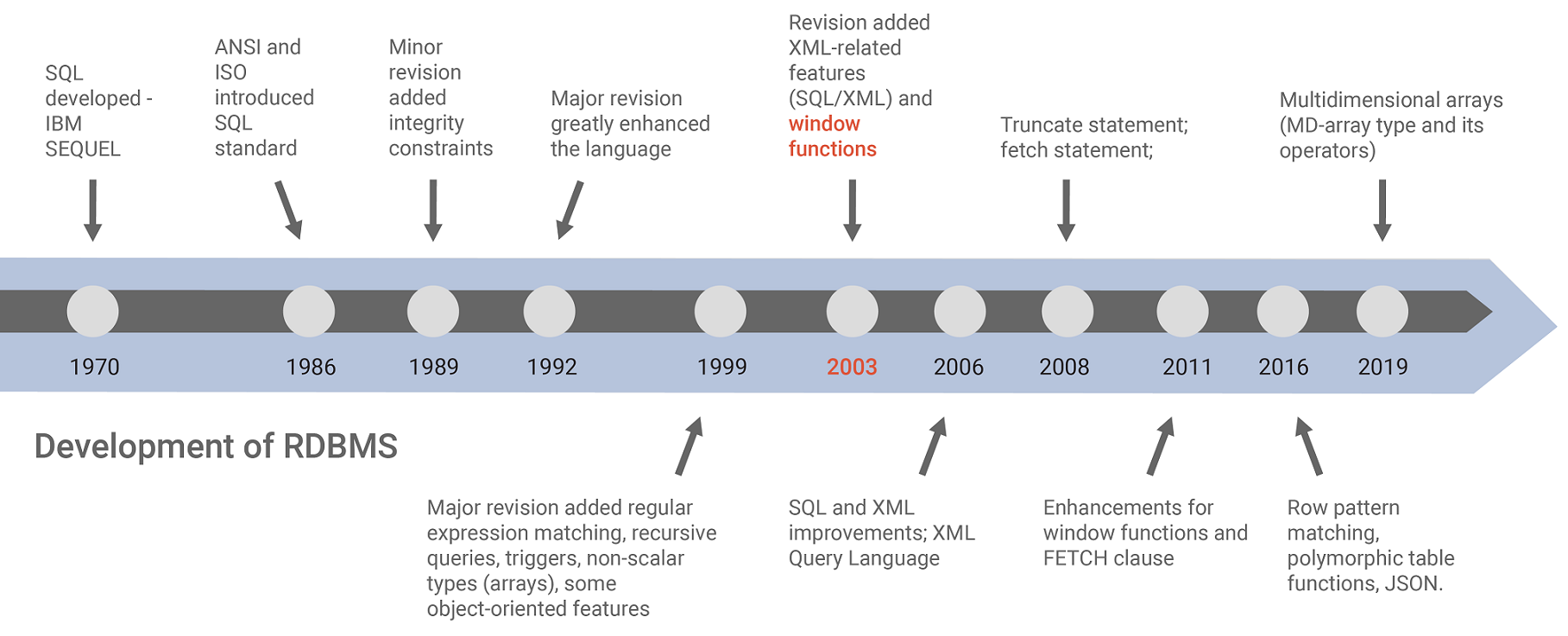History of SQL development — standardization

SQL is a famous but very old language. It was introduced in the 1970's by IBM. In 1986, the ANSI and ISO standard groups officially adopted a standard “Database Language SQL” definition (SQL-1986).

In 1992, a major revision (SQL- 92) of the initial standard was made. It’s the standard SQL we use today. That’s right – it’s been 28 years since SQL-92 was officially adopted. It’s basic concepts (SELECT, WHERE, etc.) are familiar, no matter if you’re using Oracle, DB2, MySQL, PostgreSQL, or some other database. In each DBMS, you’ll write similar SQL statements because of that same standardization.

SQL’s basic concepts are old, but they’re very useful. I’d say they are universal, as they work regardless of the industry you’re applying them to. To learn SQL, or indeed to grow as an analyst, you’re gonna have to learn these concepts.

Still, the computing world has changed a lot since 1992. Surely SQL has evolved since then? Yes, SQL evolved  and new revisions of the standards were made. The 2000’s saw the beginning of modern SQL, a concept that goes beyond basic statements. In 2003, SQL window functions were introduced. Let’s talk about the benefits of SQL window functions and why knowing them is a good thing.

First, though, let’s review something that newbies often confuse with window functions:  SQL aggregate functions.

### A Refresher on Aggregate Functions

If you are familiar with traditional SQL, then you’ve probably used aggregate functions, which allow you to perform calculations across sets of rows and get a single output row or result. For example, maybe you calculated some totals or averages over a set of rows or maybe you counted the number of rows per category. In that case, you’ve used at least some of SQL’s aggregate functions: `SUM()`, `AVG()`, `MIN()`, `MAX()`, and `COUNT()`. They are often used with the GROUP BY and HAVING clauses within SELECT statements.

Let’s see one example: how we can calculate the average price per group of rows using GROUP BY.

We are going to use Forex data on currency exchange rates. Here is our is our input table:

tickerdatetimeclose
GBPUSD2019-07-23 14:00:001.24438
GBPUSD2019-07-23 14:01:001.24454
GBPUSD2019-07-23 14:02:001.24455
GBPUSD2019-07-23 14:03:001.24461
GBPUSD2019-07-23 14:04:001.24487
GBPUSD2019-07-23 14:05:001.2448
EURUSD2019-07-23 14:00:001.11633
EURUSD2019-07-23 14:01:001.11617
EURUSD2019-07-23 14:02:001.11627
EURUSD2019-07-23 14:03:001.11636
EURUSD2019-07-23 14:04:001.1163
EURUSD2019-07-23 14:05:001.1162

Currency exchange rates — CURRENCYTRADE table

The table contains closing prices for GBP–USD and EUR–USD currency exchanges. In this example, we are using six closing prices for each currency pair. From that data, we are going to calculate the average closing price for each currency pair (GBPUSD, EURUSD) separately.

Here is the SELECT statement that calculates the average closing price for each currency pair:

`select ticker,avg(close) as average_price from CURRENCYTRADE group by ticker;`

The image below shows the result on the right: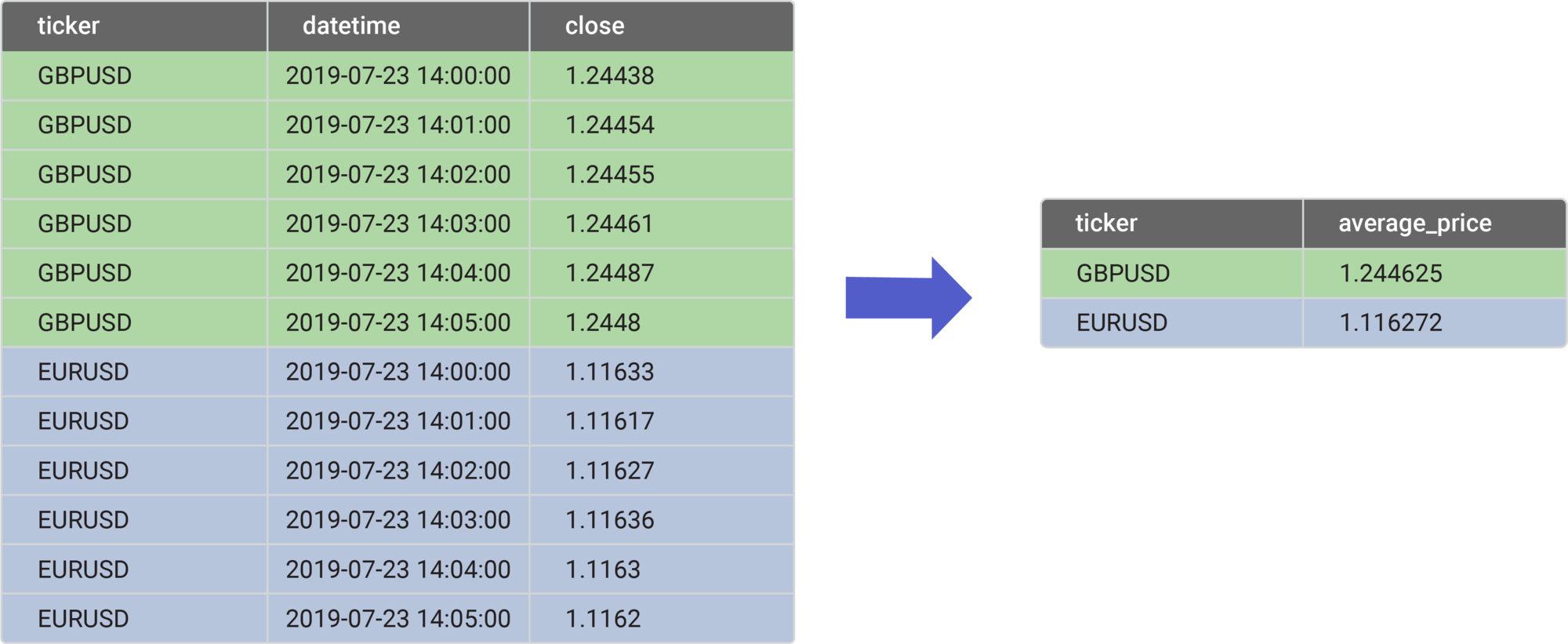The result is presented as a single row output for each currency pair. Calculations (the average value for each pair) were made on six rows for each pair. This is a simple example of an aggregate function.

Now, let’s dive into window functions.

### What Is an SQL Window Function?

Are SQL window functions similar to aggregate GROUP BY syntax? I like to think so. Like an aggregate function used with a GROUP BY clause, a window function also performs calculations across a set of rows. However, the result of a window function is not presented as a single output row per each group; that is, rows are not collapsed in the resulting table. Instead, each row from the input table is returned.

The difference between an aggregate function and a window function in SQL is simple. An aggregate function collapses all the rows into a single result, which means you lose access to the individual rows. A window function allows access to each row in the defined window. This is shown in the image below: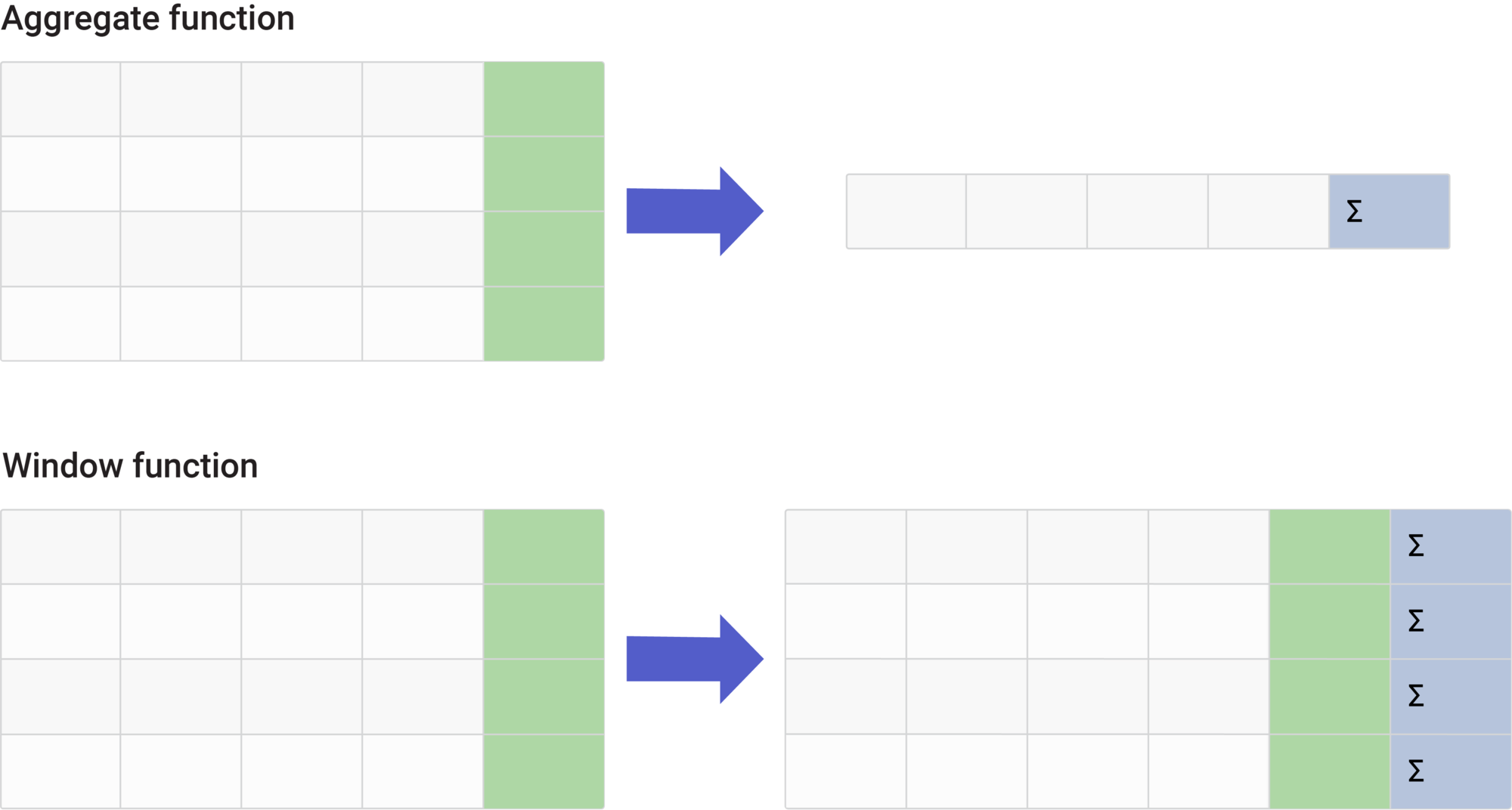Difference between SQL aggregate and window functions

To learn SQL window functions, I recommend the interactive Window Functions course at LearnSQL.com.

Let's get back to our example. If you run this part of the code ...

```select *,avg(close) OVER(PARTITION BY ticker) AS average_closing from CURRENCYTRADE ;
```

… the average closing price for each currency pair will be assigned to each row from the `CURRENCYTRADE` table.

The result will be something like this: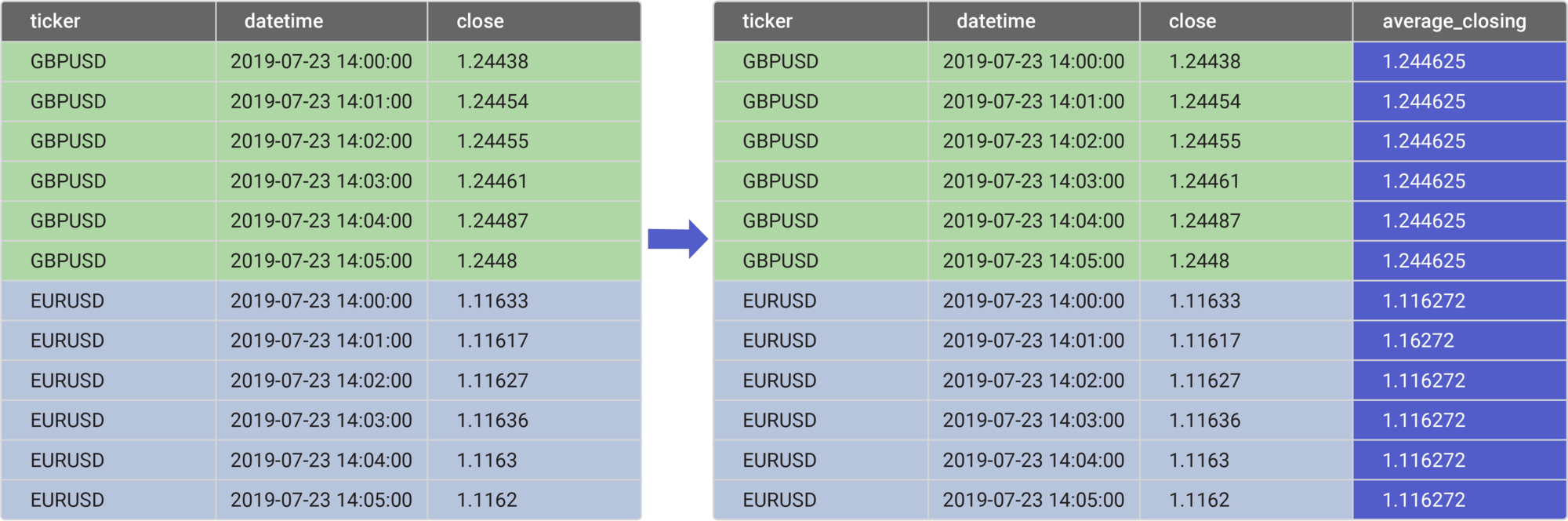As you can see, the window function did not group the output into a single output row per currency pair group. Instead, each row now contains additional info: the average closing price for the appropriate currency pair. This can be very useful, as many analyses will require some additional info for each row while still keeping all columns from the initial data set.

### Window function syntax

In our last window function example, we used some special keywords like OVER() and PARTITION BY:

```select *,avg(close) OVER(PARTITION BY ticker) AS average_closing from CURRENCYTRADE ;
```

Those are the main keywords that define a window function. Here is a short explanation:

1. OVER denotes that this is a window function. Inside the OVER clause, we can have PARTITION, ORDER BY, and other window frame clauses that tell us how the window is framed (i.e. the groups and how they are ordered). Although we didn’t use the window frame (ROW or RANGE) clause in our example, keep in mind that you can use either inside the OVER clause to specify frames within partitions.
2. PARTITION tells us how the data is grouped or framed. It is placed inside the OVER clause. In our example, we calculated the average price for each currency pair; thus, we partitioned our window by the column ticker.
3. ORDER BY (which we didn't use in our example) is often used to determine the order of rows inside each frame.
4. ROW or RANGE is used if we want to further limit the rows within the partition. We do this by specifying the starting and ending points within the partition. No matter which of these two clauses you employ, it must be used with ORDER BY. The syntax looks like this:
```[ROWS | RANGE] BETWEEN  AND
```

The <starting_row> is denoted by one of the following:

• UNBOUNDED PRECEDING: The window starts in the first row of the partition.
• CURRENT ROW: The window starts in the current row.
• <unsigned integer literal> PRECEDING or FOLLOWING.

And the <ending_row> uses one of the following:

• UNBOUNDED FOLLOWING: The window ends in the last row of the partition.
• CURRENT ROW: The window ends in the current row.
• <unsigned integer literal> PRECEDING or FOLLOWING.

You can find more examples of window frame clauses here.

Don’t worry if you don’t feel comfortable with this syntax. Practice will help; I can recommend LearnSQL.com’s Window Functions course, which has a lot of good info.

Now that you've learned how you can use SQL analytic functions in your queries, the next step is to take a look at the types of window functions available in SQL. So far, we’ve only shown how AVG() ( an aggregate function) can be used as a window function. Let’s see what the other functions can do.

### Window function types

There are three main types of window functions:

• Aggregate window functions: `AVG()`, `MIN()`, `MAX()`, `COUNT()`, `SUM()`. These functions are to calculate average, minimum, or maximum values, the total number of rows, or the total sum inside each defined frame. Aggregate window functions return a single value for each row from the underlying query.
• Ranking window functions: `RANK()`, `ROW_NUMBER()`, and similar. Ranking window functions are used to rank rows inside each frame. For example, `RANK()` will rank a value in a group of values. The ORDER BY expression in the OVER clause determines the rank value. Each value is ranked within its partition. Rows with equal values for the ranking criteria receive the same rank.

Let’s have another example of a ranking window function. `ROW_NUMBER()` determines the ordinal number of the current row within its partition. Once again, the ORDER BY in the OVER clause determines this number. Each value is ordered within its partition.

• Value window functions: `LAG()`, `LEAD()`, `FIRST_VALUE()`, `LAST_VALUE()`. These functions really help in reporting and summarizing data. The `LAG()` and `LEAD()` window functions return the value for the row before (`LEAD()`) or after (`LAG()`) the current row in a partition. If no row exists, a null is returned. Similarly, the `FIRST_VALUE()` / `LAST_VALUE()` window function returns the value of the specified expression for the first (or last) row in the window frame.

We’ve already seen an example of how to use aggregate window functions, so you can understand why they’re useful in data analysis. Actually, all three types of SQL window functions are used frequently in complex analyses. They are a great feature in SQL.

Need a real-life example? Well, suppose you’re in a Forex trading business. Quite often when selling or buying positions, you examine the closing price of the previous minute or the previous hour; for that, you’d use the `LAG()` function. You could also rank your closing prices using ranking window functions inside a specific time frame window. Or you could find the initial or final closing price by using value window functions.

### Using SQL window functions: LAG()

Let's go deeper into using SQL analytic functions in real life. For each row, let’s see the closing price of the previous row. We’ll use a ranking window function:

```select *,LAG(close) OVER(PARTITION BY ticker ORDER BY datetime) AS previous_close from CURRENCYTRADE;
```

We are using LAG(), which returns the value from the previous row. Here, the OVER denotes that this is a window function in which we are grouping rows per currency pair. Because we are using LAG(), we also need an ORDER BY clause to sort the data inside each frame before assigning closing prices from the previous row. We are ordering the data by the datetime column, which means that we’ll have the closing price for the previous minute in each current row. The image below shows how the output looks: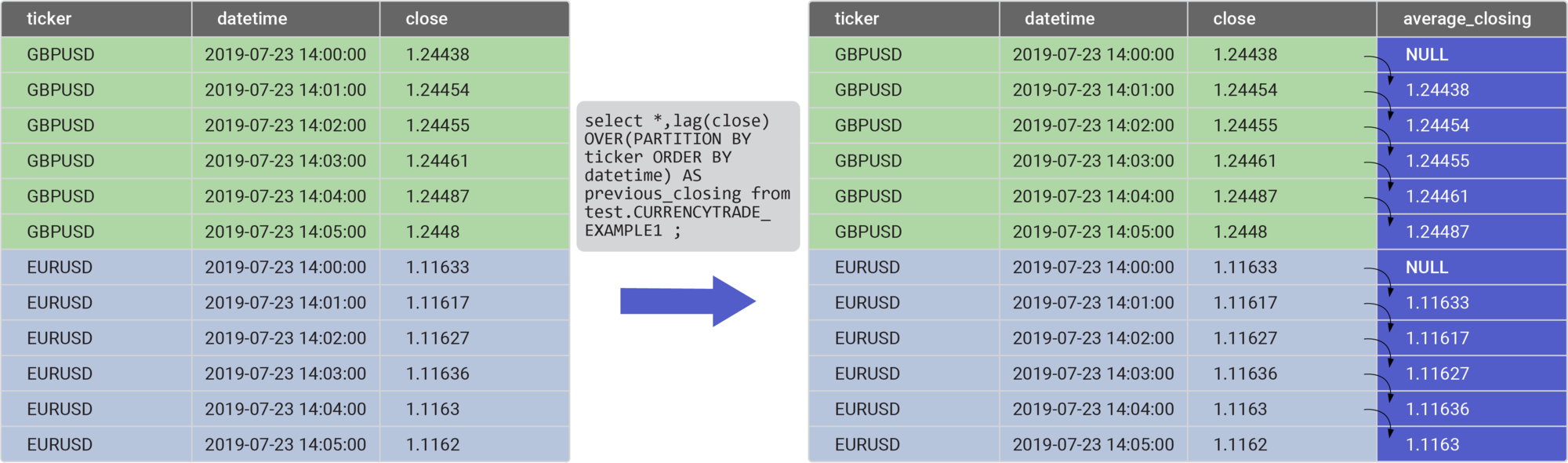Window lag function

This code is simpler and easier to maintain. That’s a major benefit of using window functions. Indeed, they have a lot of benefits, as we’ll see.

### Benefits of Using SQL Window Functions

Window functions are useful when you do not need to collapse rows in the resultset, that is, group the result data in a single output row. Instead of a single output row, a single value for each row from the underlying query is returned. That is the main benefit, if you ask me.

Some of the other benefits of SQL analytic functions include:

• Window functions allow you to gather both aggregate and non-aggregate values at once. This is because for each row value returned, there is no grouping or collapsing of that row. You can keep all the columns from each row plus add additional values calculated by the window function. This is a major advantage when you need both aggregate and non-aggregate values in one table.
• Their syntax is simple, and it is easier to maintain the code in production. Imagine how much time it would take you to implement the equivalent to the `LEAD()`, `LAG()`, or `RANK()` function using traditional SQL. Or to just assign averages to each row without window functions! You’d need to first use the aggregate GROUP BY function, followed by a LEFT JOIN with the original input data table. Your code would be more complicated and harder to maintain. Here is an interesting article that shows how one example can be solved with both cursors and SQL analytic functions. The analytic function code is cleaner and simpler, don’t you think?
• You can easily assign to a current row a value from a previous row or a successive row. In some databases this is actually a much faster option than using a solution with the cursor or a correlated subquery. Such codes are more complex and harder to maintain. Here is a nice article that compares performance (window functions vs. cursor vs. subquery) in a MS SQL database. Cool, right?